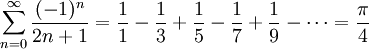Name: ___K_E_Y__________________ Student ID: __________________________
1. Name the five steps to top-down
problem solving as described in the text.
(It's okay if you can't remember the exact wording; the concepts are more important.) 

 Write______ Apprehend__ Design_____ Execute____ Scrutinize_
2. Name the five computer program flow abstractions described in the text. 

 Sequence___ Selection__ Repetition_ Composition Parallelism
3. Write "yes" or "no" next to each of the following six strings to indicate if it is a legal Python identifier. 
 numApples __yes_ 21st_birthday __no__ prime-factor __no__ for __no__ _illegal __yes_ L337_hAX0rz __yes_
4. Evaluate each of the following Python expressions, or if it gives an error, indicate why. 
 (5 % 12 == 2) or False and not False ___False_________________ 2 ** 3+16.2 // 5 ___11.0__________________ 0!=-0 and 0-0/0<0 ___False_________________ 'pi' + 2*'z' + 'a' ___'pizza'_______________ 2*range(3) ___[0, 1, 2, 0, 1, 2]____
5. The following loop was intended to count down from ten to one, then print "Blast off!". What is wrong with the loop? How would you fix it? 
```counter = 10.0
while counter != 1.0:
print int(counter),
counter -= 1.0
print "Blast off!"

```
The loop finishes before printing '1': the output is
`	'10 9 8 7 6 5 4 3 2 Blast off!'`
A quick fix would be to make the while loop condition be:
```while counter > 0:
```
6. Recall that in Python int/float/bool parameters are essentially passed by value, and lists/dictionary parameters are essentially passed by reference. What is printed by the following block of Python code? 
```def double_me(x):
x *= 2
y = 3.0
z = range(3)
double_me(y)
double_me(z)
print 'y =', y, ', z =', z
```
```y = 3.0 , z = [0, 1, 2, 0, 1, 2]
```
The variable y points to an immutable object (the float 3.0) and is roughly pass-by-value, so it is not affected by double_me(). The variable z points to a mutable object (the list range(3)) and is roughly pass-by-reference, so it is changed by double_me(). The real scoop on Python parameter passing is call-by-object and is a bit more complicated; it has to do with Python's object-oriented roots.
7. Leibniz' formula for calculating pi is an infinite series:In the space below the next problem, write pseudocode for a function procedure leibniz() that returns an estimate of pi. A parameter should be used to tell the function the maximum value of n to use in the sum. For example, leibniz(3) should add terms up to and including n==3. 

Here's what I came up with:

• The parameter to the function is the maximum value of n to use.
• Initialize the sum to 0.
• For n from 0 up to and including the maximum value:
• Calculate (-1)**n / (2n+1) and add it to the sum
• Multiply the sum by 4 and return the result
8. On a separate sheet of paper, write a complete Python program, declaring the procedure leibniz(). The body of the program should invoke leibniz() a few times to test it out, using a different number of terms of the infinite series. (You could also let the user interactively specify the parameter to leibniz().) 

Docstrings (including preconditions) are required for your whole module and for each function. You are not required to comment your code on this exam, however if your code is incorrect, your comments may earn you partial credit if they show good design thinking. Little partial credit will be given for uncommented incorrect code.